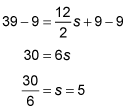##### GED Mathematical Reasoning Test For Dummies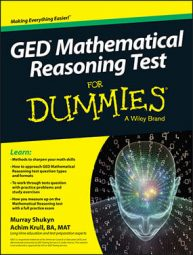The GED Mathematical Reasoning test is likely to ask a few questions that involve volume and surface area. When taking the computer version of the test, a formula sheet is supplied, but you will have to know how to apply these formulas.

The following sample questions ask you to find the diameter of a can based on its volume and height, and the slant length of a pyramid, given its side length and surface area.

## Practice questions

1. If a can is designed to contain 24 cubic inches of coconut water and has to be 6 inches tall to fit on the shelves in a store, what would be its diameter in inches?

A. 1.72

B. 1.27

C. 1.79

D. 2.26

2. A pyramid has a square base with one of its sides being 3 feet long and a total surface area of 39 square feet. What is the slant length of one of its sides?

1. D.

The can would be 2.26 inches in diameter. Substituting in the formula for the volume of a right cylinder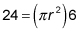divide both sides by 6 to simplify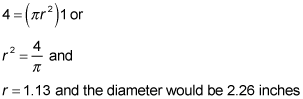2. 5 feet

To answer this question, use the surface area formula for a pyramid,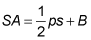and plug in the values you have: The area of the base is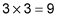the perimeter is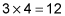and the surface area is 39 square feet, so you have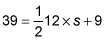Do the math: